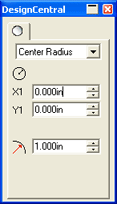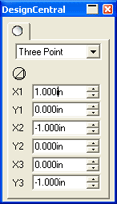### Circles

For circles, you can adjust the following parameters:

 Center Radius Sets the X,Y coordinates of the center and the radius that determine the circle's shape. Two Point Sets the X,Y coordinates of the two points that determine the circle's shape. Three Point Sets the X,Y coordinates of the three points that determine the circle's shape.Center RadiusTwo PointThree Point